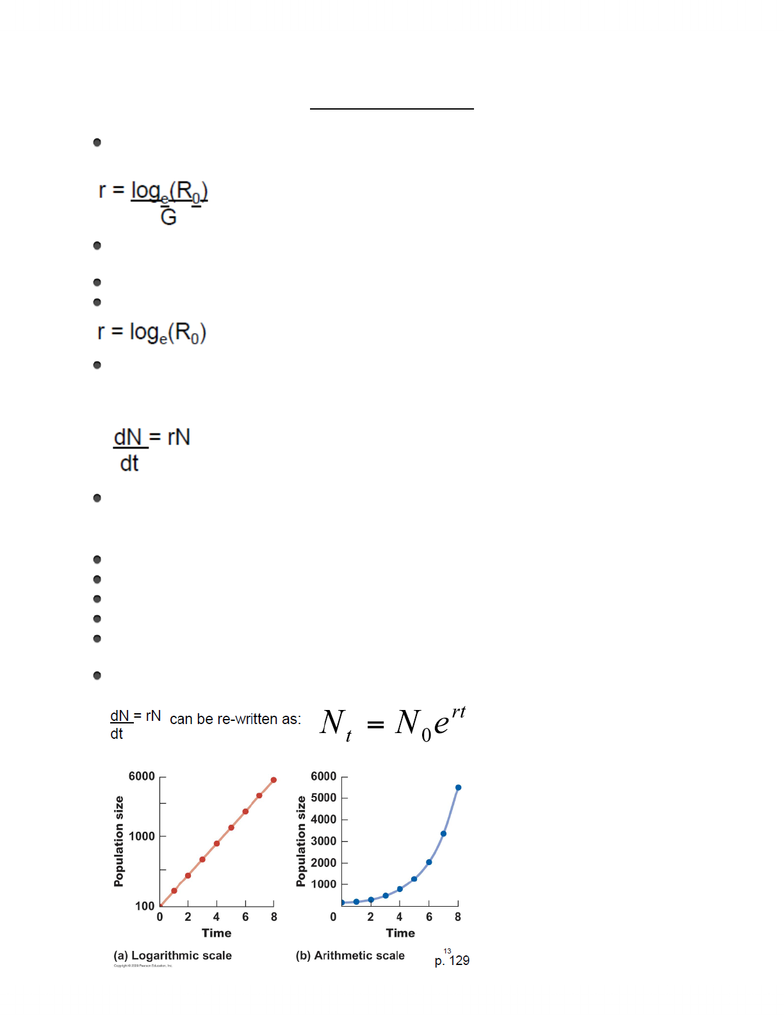# BIOL 215 Lecture Notes - Lecture 14: Leslie Matrix, Carrying Capacity, Logistic Function

19 views4 pages
School
Department
Course
ProfessorBIOL215 Lecture 14 Notes
The rate of increase in a population that we are obtaining is the "instantaneous rate of increase" - at
one specific point in time, how the population is increasing. The equation is:
In the case of an annual species that becomes the annual rate of increase (reproductive rate for an
annual species is equal to the rate of increase per year of that population; is the simplest case)
In the simplest case, the annual species occurs when G=1 (one generation time)
The instantaneous rate of increase is calculated by:
We can use the rate of increase to build another measure of population growth or to develop an
equation that will allow us to predict a population over time. We call this the geometric growth,
calculated by:
where r is intrinsic capacity for increase in a given environment
Under a geometric growth:
Any population subject to fixed schedule of natality and mortality grows in geometric way
Geometric growth dictate fixed and unchanging age distribution, the stable age distribution
If the rate of increase is less than zero, the population will decrease
If the rate of increase is zero, the population will stay stable (same)
If the rate of increase is one, the population will double
If the rate of increase is greater than zero, the population will increase
In geographic model, the population on the log scale increase always of the same amount per year or
per time interval because r is fixed (therefore, linear curve)
On an arithmetic scale, you have an exponential growth (a population that will increase and as you
have more and more individuals, the increments will increase faster and faster)
Unlock document

This preview shows page 1 of the document.
Unlock all 4 pages and 3 million more documents.

# Get access

\$10 USD/m
Billed \$120 USD annually
Homework Help
Class Notes
Textbook Notes